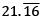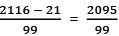Courses

# Test: Number System (Easy)

## 15 Questions MCQ Test Class 11 | Test: Number System (Easy)

Description
This mock test of Test: Number System (Easy) for CAT helps you for every CAT entrance exam. This contains 15 Multiple Choice Questions for CAT Test: Number System (Easy) (mcq) to study with solutions a complete question bank. The solved questions answers in this Test: Number System (Easy) quiz give you a good mix of easy questions and tough questions. CAT students definitely take this Test: Number System (Easy) exercise for a better result in the exam. You can find other Test: Number System (Easy) extra questions, long questions & short questions for CAT on EduRev as well by searching above.
QUESTION: 1

### What is the Place Value and Face Value of 7 in 78654?

Solution:

Face Value of any number remains the same irrespective of the place, for 7 it is 7

Place Value = Place Multiplier x  Face Value

= 10000 x 7
= 70000

QUESTION: 2

### In which one of the following is the Place Value of 9 highest?

Solution:

Option A :

• Place value of 9 = 9 x 1000 = 9000

Option B :

• Place value of 9 = 9 x 100 = 900

Option C :

• Place value of 9 = 9 x 1 = 9

Option D :

• Place value of 9 = 9 x 10 = 90
QUESTION: 3

### What is the Base of Hexadecimal Number System?

Solution:

► 8 is the Base of Octal Number System
► 16 is the Base of Hexadecimal Number System
► 2 is the Base of Binary Number System
► 10 is the Base of Decimal Number System

QUESTION: 4

Which of the following is a Real Number but not a Rational Number?

Solution:

► −√4 = −2 is a Real Number as well as a Rational Number
► −√3 is a Real number and an irrational number
► √−4 is an imaginary number

QUESTION: 5

Convertin p/q form

Solution:

► All the digits written once = 2116
► All the digits without Bar written once = 21
► Number of digits with bar after decimal = 2
► Number of digits without bar after decimal = 0
► Rational form =QUESTION: 6

√16 will not fall under which of the following categories?

Solution:

► √16 = 4, which is a natural number, rational number as well as a real number.

QUESTION: 7

(16a + 6) will fall under which of the following categories, a is an Integer

Solution:

(16a + 6) will always be even, whether the a is odd or even.

For a = 1 (odd)

(16 * 1) + 6 = 16 + 6

= 22 (even)

For a = 2 (even)

(16* 2) + 6

= 32 + 6

= 38 (even)

QUESTION: 8

If (7a - 3) is odd then 'a' would be, a is an Integer

Solution:

As it is given, 7a - 3 is odd

so, 7a = odd +3 = (even)

assume x and given , a is an integer

so, 7a = x, x should be completely divisble by 7

so, a = x/7 = (even/odd) = even

QUESTION: 9

What is the smallest possible positive Integer?

Solution:

Option A: 0 is an integer but not positive(it is neutral).
► Option B: 1 is an integer also positive and the smallest.
► Option C:  0.5 is not even an integer.
► Option D:  2 is an integer as well as positive but not the smallest.

QUESTION: 10

Which of the following is a prime number?

Solution:

► Option A: 93 is divisible by 3
► Option B: 97 is not perfectly divisible by any number.
► Option C: 95 is divisible by 5
► Option D: 91 is divisible by 7

QUESTION: 11

1837 × 1712 × 1839 will be

Solution:

We do not need to do the multiplication in this question.
► 1837 is Odd
► 1712 is Even
► 1839 is Odd

Odd x Even x Odd = Even

Only option 1 is Even rest all are Odd.

QUESTION: 12

Largest 3 digit composite number is

Solution:

► 999 is the Largest composite number, it is divisible by 1, 3, 9, 111, 333, 999.

QUESTION: 13

What is the difference between 517th Whole number and 516th Natural Number?

Solution:

► 517th Whole number = 516
► 516th Natural Number = 516

• 516 - 516 = 0
QUESTION: 14

Smallest Composite numbers is______.

Solution:

► Prime and Composite are properties of Natural numbers.
► 0 is not a natural number.
► 1 is neither prime nor composite.
► 2 is a prime number divisible by 1 and 2 only.
► 4 is a composite number divisible by 1,2 and 4. Smallest among all.

QUESTION: 15

How many pairs of consecutive prime numbers are there?

Solution:

► Only pair of consecutive prime numbers is (2, 3)